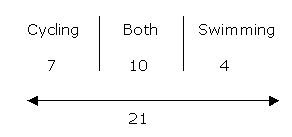This blog is managed by Song Hock Chye, author of Improve Your Thinking Skills in Maths (P1-P3 series), which is published and distributed by EPH.

## Tuesday, September 09, 2008

### Catholic High Sch 2006 PSLE Math Prelim Q36

There are 45 boys in a campsite. Out of these boys, 17 of them like cycling. 14 of them like swimming and 10 of them like both cycling and swimming. How many boys do not like cycling and swimming?

SolutionLike both ----- 10
Like cycling only ---- 17 – 10 = 7
Like swimming only ----- 14 – 10 = 4
(Total number of boys) like cycling, swimming or both -----
10 + 7 + 4 = 21

(Number of boys) do not like cycling and swimming -----
45 – 21 = 24

Answer: 24 boys do not like cycling or swimming.

## Friday, September 05, 2008

### Catholic High Sch 2006 PSLE Math Prelim Q29

Alan has enough money to buy either 18 pencils or 15 pens. If he buys 12 pencils, how many pens can be buy with the remaining money?

Solution

18 pencils ----- 15 pens
(divided both by 3)

6 pencils ----- 5 pens

If he bought 12 pencils, it means he bought 6 pencils less. This amount of money is equivalent to the price of 5 pens.

Answer: He could have bought 5 pens with the remaining money.

### Catholic High Sch 2006 PSLE Math Prelim Q27

Kenneth wants to rent a bicycle from Jim’s Bicycle Kiosk. The cost (C) of renting a bicycle for d hours is given by C = \$(3d + 5). Find the cost of renting the bicycle if Kenneth wants to cycle for 4 hours.

Solution

d hours ----- \$(3d + 5)
1 hour ----- \$(3d + 5)/d
4 hours ----- \$4(3d + 5)/d (Answer)

## Thursday, September 04, 2008

### Catholic High Sch 2006 PSLE Math Prelim Q26

The figure below is made up of a square and three identical semi-circles.
Calculate the perimeter of the shaded region.= (2 x 3.14 x 5 cm) + 10 cm + 10 cm
= 31.4 cm + 20 cm= 51.4 cm (Answer)

## Monday, September 01, 2008

### Common Errors and Confusion – “ovary” and “spores”

It is a bit unfortunate that both the reproductive parts of the female human and the female part of the flower have the same name, “ovary”. Because both have the same name, spelling and the same way they are pronounced, some students end up confused.

Ovary in a flower refers to the female reproductive part of the flower. The ovary in this case, will develop into the fruit after fertilization. The ovary of the flower is illustrated as shown below.Ovary in the human female on the other hand, is the reproductive part of the female where eggs are produced. The ovary in the human female is illustrated as shown below.Another confusion which is caused by the same term, is “spores”. Spores are produced by ferns and mushrooms for the purpose of reproduction. Again, because of the same name, some students get confused that mushrooms and ferns are plants. This is not correct. Ferns are plants but mushrooms are fungi. Only plants make their own food. Mushrooms feed on dead matter - eg rotting logs.

### PSLE Aggregate Score Calculation

Your individual PSLE Aggregate Score depends on your cohort’s performance and how easy or tough the paper is.

Using the individual’s score of Eng 82, MT 89, Maths 87, Science 86, two scenarios are created below.

Example 1 - where it is assumed the cohort is weak, and the paper is assumed “tough”. An average score of 55 is used, and the Standard Deviation of 20 is used.PSLE Aggregate Score is worked out to be 262.

Example 2 - Using the same individual score, but the cohort is now assumed to be stronger, and the paper is assumed to be “simple”. A higher average of 60 is used, and a higher Standard Deviation of 25 is used.PSLE Aggregate Score is worked out to be 242.

Rationale for adjustment - If the cohort is strong, it can be assumed that pupils will be able to score a higher average. Conversely, if the cohort is weak, the average of the cohort will drop.

If the paper is “simple and easy”, it is assumed that more students will be able to score "A*"s and "A"s. Hence, an “easy” paper will widen the gap between the brighter and average pupils, giving a larger Standard Deviation.

Likewise, if the paper is "tough", it is assumed that fewer students will be able to score "A*"s and "A"s, narrowing the gap between the brighter and average pupils, giving a smaller Standard Division.

Note - For those who are using the PSLE Aggregate score calculator, using a low average and low Standard Deviation would create a "most liberal scenario" where the PSLE Aggregate Score Calculator will show a high result. Using a high average and high Standard Deviation would create a "most conservative scenario", where the PSLE Aggregate Score will show a much more conservative result.

Related posts
How PSLE Aggregate Score is calculated
Get a free copy of your PSLE Aggregate Score Calculator